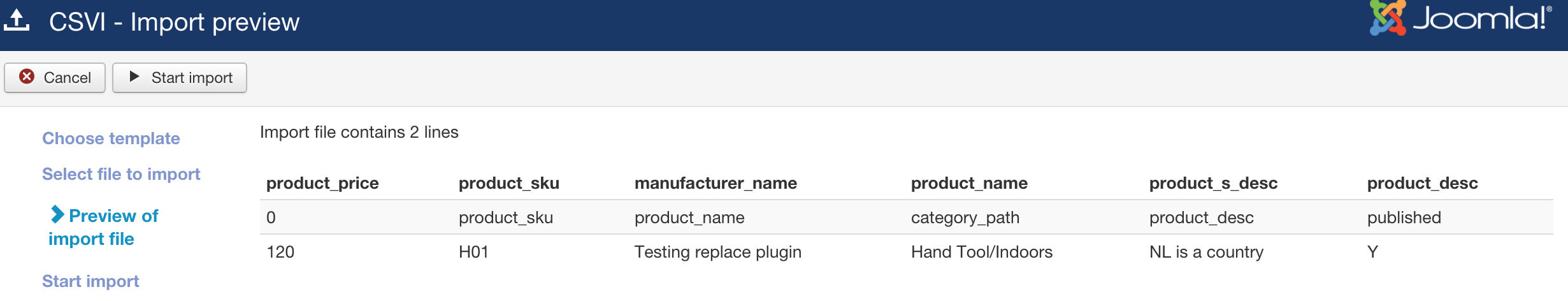# RO CSVI

## Margin plugin

The Margin plugin let's you set a margin to the product price (for example) and imports the new price according to the value set.

From Components -> RO CSVI ->Templates tab -> Rules, Click New to add a new rule for margin plugin.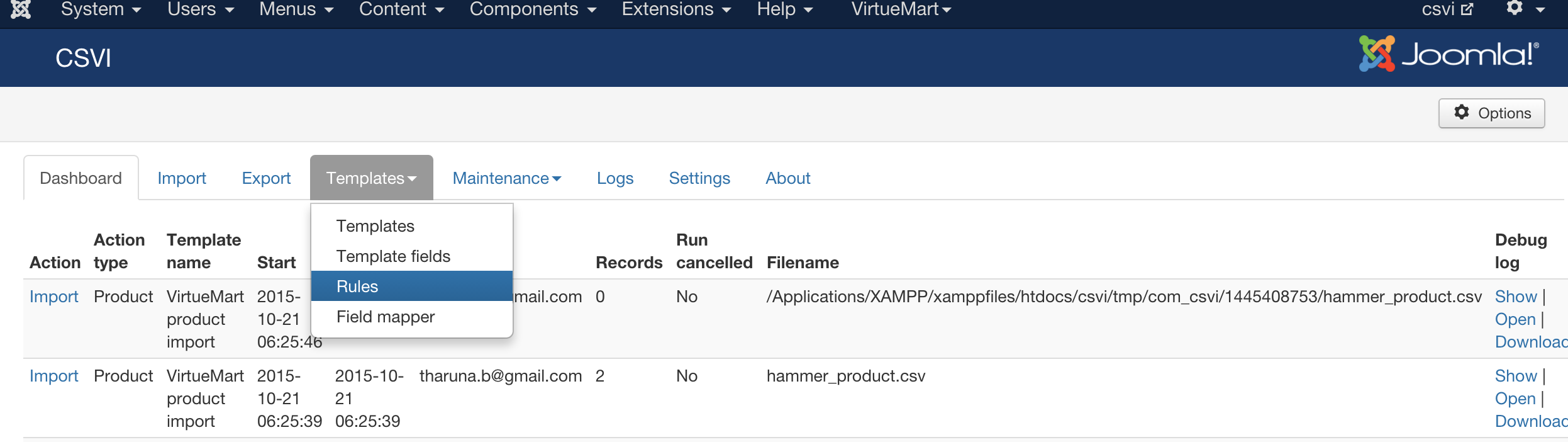For example to create a rule to set 20% margin for product_price field of a virtuemart product.

1. Give a Descriptive name for the rule in name field.
2. Select Action as Import.
3. Select Plugin as RO CSVI margin.
4. Click on load plugin button.

You will now see three new fields appears

1. Value type field to select how the margin is calculated either percentage or value.
2. Margin field to set how much percentage or value to be added, For example here its 20.
3. Operation type used to calculate the margin.

### Example

Here is an example on how to create a rule using margin plugin for a product_price field in virtuemart.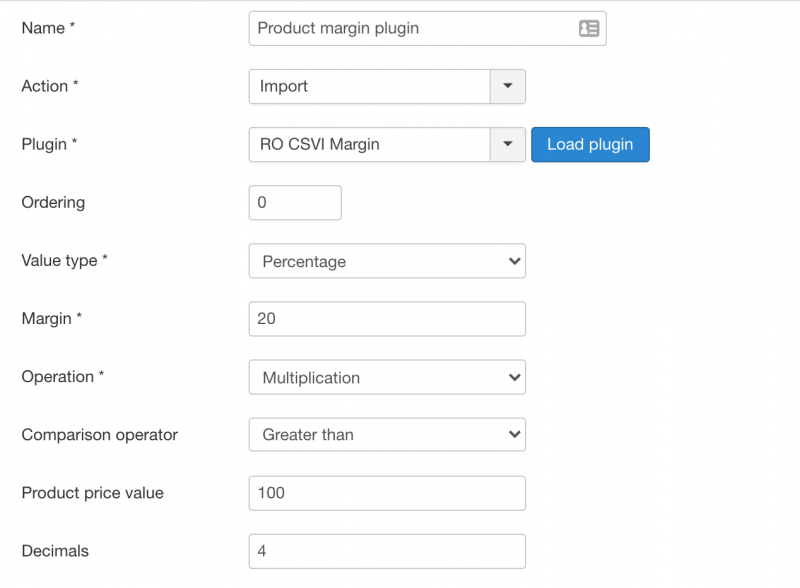Explanation on the fields

Value type

Take the percentage of value or the value itself.

Margin

Value to be taken for percentage, with the example in the above screenshot, 20% is taken as margin.

Operation

The type of arithmetic operation to be used for calculating the margin. This example uses Multiplication. So for 100 as product_price, margin as 20% with the rule applied, final product_price would be 120 on import.

Comparison Operator

Compare product_price value before applying the margin.

Product price value

The value to compare product_price with. With the above settings in the screenshot, product_price is compared if it is greater than 100 before applying the margin.

Decimals

The number of decimals to be taken after the margin calculation. In this case 4 decimals are taken.

Now set this rule to template fields to use the margin plugin like below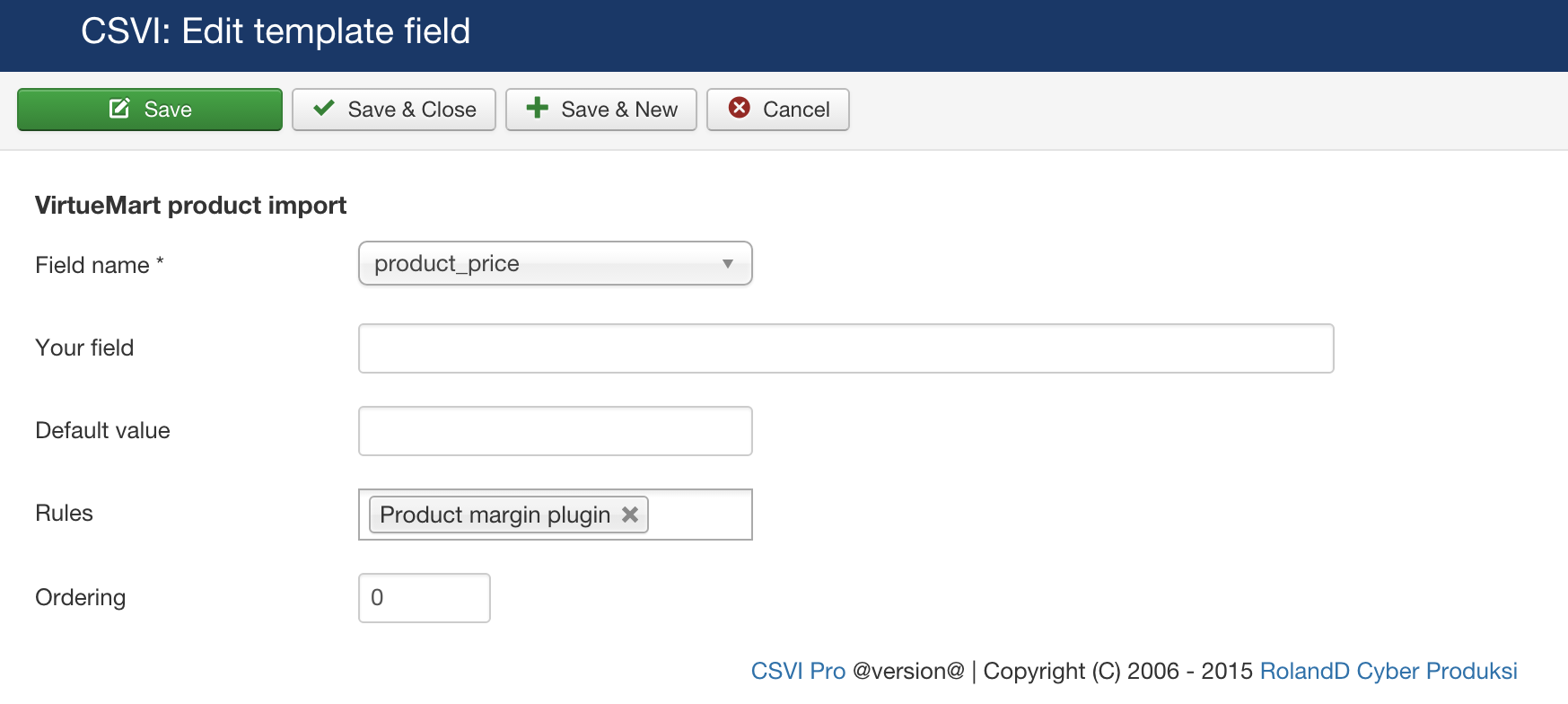When the fields are set with rules you can see a small fire icon next to the field name in Template fields tab.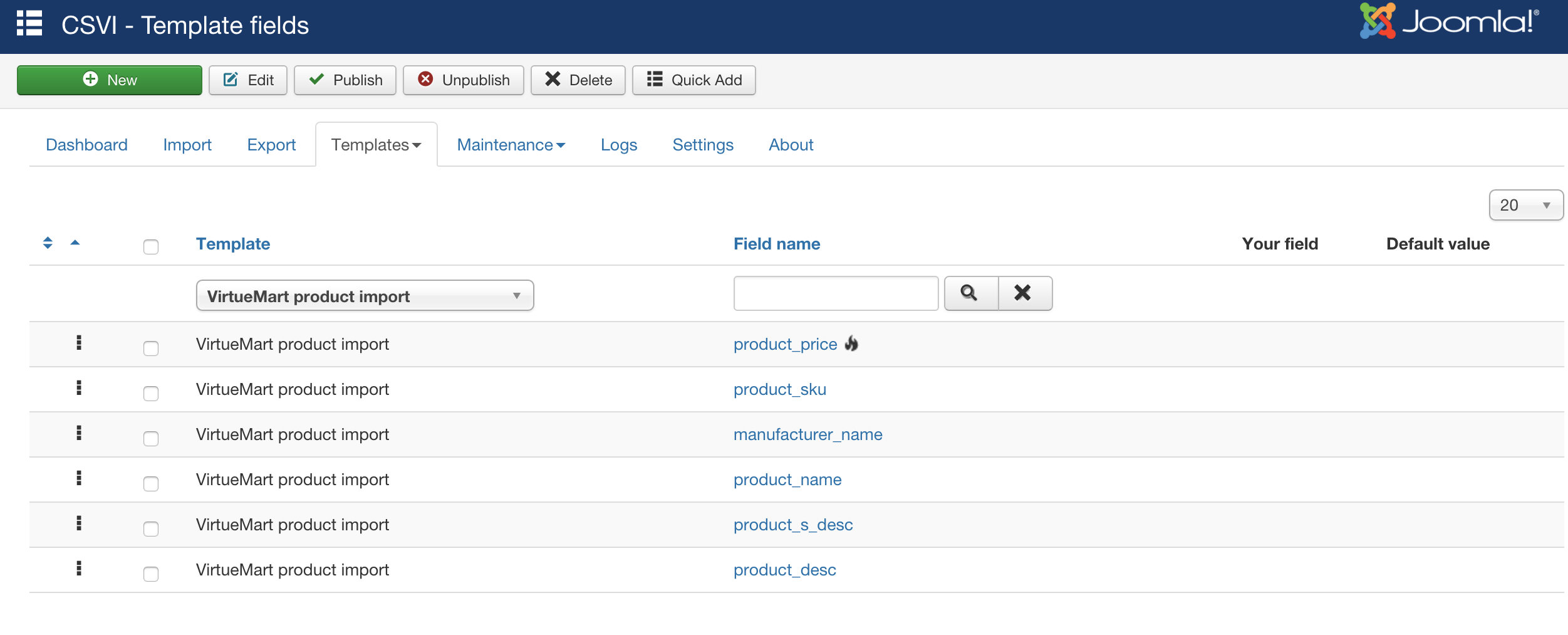Important Note :

•  Make sure that  product_price (example used in this template) are added in template fields list.
• Use file for configuration option in templates->template name->file tab set to No.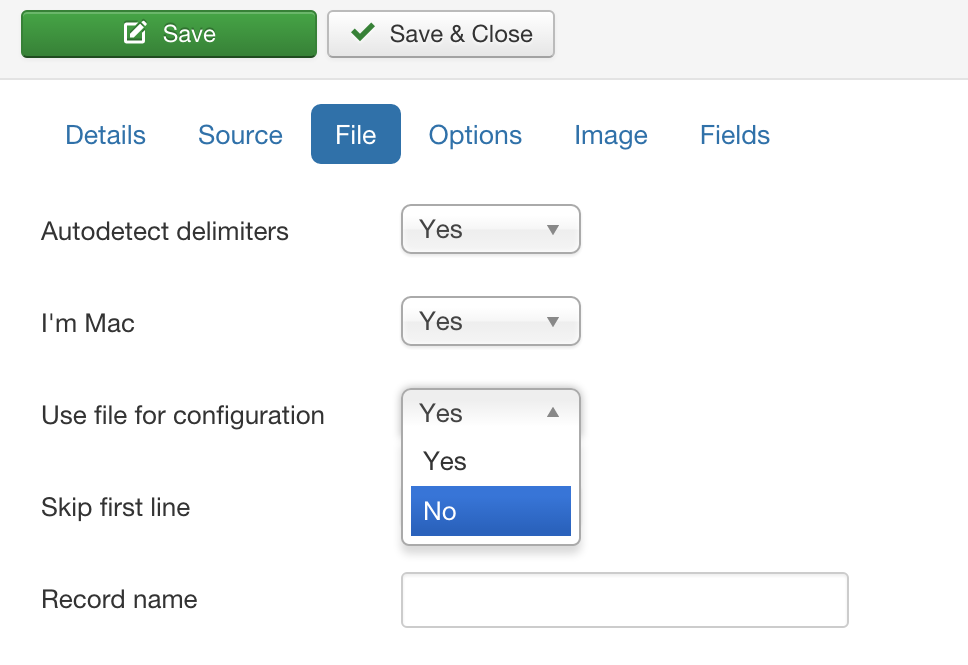Now that all rules and fields are set, CSVI will automatically calculate the product_price according to the new margin set. Say here for an example the product_price in CSV file has been given as 100. While import CSVI will calculate the price and show as below.